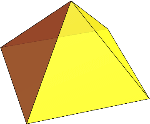Search IntMath
Close

# Understanding 3D Square Pyramid in Geometry## What is a Square Pyramid?

A 3D Square Pyramid is a three-dimensional geometric shape that consists of four triangular faces and one square base. It is a type of polyhedron and is a special type of pyramid, with the base being a square instead of a triangle. All the faces meet at the apex of the pyramid, which is the highest point of the pyramid.

## Faces of a Square Pyramid

A 3D Square Pyramid has five faces: four triangular faces and one square base. The four triangular faces are all connected to one another and meet at the apex of the pyramid. The square base lies in the bottom of the pyramid, and the four sides of the square base are all connected to the four triangular faces.

## Volume of a Square Pyramid

The volume of a 3D Square Pyramid is equal to one-third of the base area multiplied by the height of the pyramid. This means that if the square base of the pyramid has a length of l and a width of w, and the height of the pyramid is h, then the volume of the pyramid is equal to: V = (l * w * h) / 3

## Calculating the Area of a Square Pyramid

The area of a 3D Square Pyramid can be calculated by adding the area of the four triangular faces together with the area of the square base. The area of the four triangular faces can be calculated by using the formula: A = 1/2bh, where b is the base and h is the height of the triangle. The area of the square base can be calculated by using the formula: A = l * w, where l is the length and w is the width of the square base.

## Practice Problems

Let's test your understanding of 3D Square Pyramids in geometry with some practice problems.

1. Find the area of a 3D Square Pyramid with a base length of 4 and a base width of 6, and a height of 8.

Answer: The area of the 3D Square Pyramid is 208.

2. Find the volume of a 3D Square Pyramid with a base length of 5 and a base width of 6, and a height of 7.

Answer: The volume of the 3D Square Pyramid is 70.

3. Find the area of a 3D Square Pyramid with a base length of 10 and a base width of 10, and a height of 8.

Answer: The area of the 3D Square Pyramid is 400.

4. Find the volume of a 3D Square Pyramid with a base length of 8 and a base width of 8, and a height of 6.

Answer: The volume of the 3D Square Pyramid is 192.

5. Find the area of a 3D Square Pyramid with a base length of 9 and a base width of 9, and a height of 5.

Answer: The area of the 3D Square Pyramid is 225.

6. Find the volume of a 3D Square Pyramid with a base length of 7 and a base width of 7, and a height of 4.

Answer: The volume of the 3D Square Pyramid is 98.

## Summary

In this article, we have discussed 3D Square Pyramids in geometry. We discussed what a 3D Square Pyramid is, its faces, and how to calculate its volume and area. We also provided some practice problems that can help you understand this concept better. We hope this article was helpful in understanding 3D Square Pyramids in geometry.

## FAQ

### Why is it called a square pyramid?

A square pyramid is called so because it has a square base. All the other faces of the pyramid are triangles.

### What is a pyramid draw and explain the parts of a square pyramid?

A pyramid is a three-dimensional shape with a square or polygonal base and four triangular faces that meet at a point. The base of the square pyramid is a square and the other four faces are triangles. The point at which the four triangles meet is called the apex.

### How do you explain a pyramid?

A pyramid is a three-dimensional shape with a square or polygonal base and four triangular faces that meet at a point. The base of the pyramid is the largest face, and all other faces are triangles that meet at the apex, which is the top of the pyramid.

### What does a square pyramid look like?

A square pyramid looks like a regular pyramid with a square base and four triangular faces that meet at a point at the top. The base of the pyramid is the largest face, and all other faces are triangles that meet at the apex.

## Problem SolverThis tool combines the power of mathematical computation engine that excels at solving mathematical formulas with the power of GPT large language models to parse and generate natural language. This creates math problem solver thats more accurate than ChatGPT, more flexible than a calculator, and faster answers than a human tutor. Learn More.